Courses
Courses for Kids
Free study material
Offline Centres
More

# NCERT Exemplar for Class 6 Science Solutions Chapter 10 Motion and Measurement of DistancesLast updated date: 26th Nov 2023
Total views: 423.6k
Views today: 11.23k

## Class 6 Science NCERT Exemplar Solutions Chapter 10 Motion and Measurement of Distances

Free PDF download of NCERT Exemplar for Class 6 Science Chapter 10 Motion and Measurement of Distances solved by expert Science teachers on Vedantu as per NCERT (CBSE) Book guidelines. All Chapter 10 Motion and Measurement of Distances exercise questions with solutions to help you to revise the complete syllabus and score more marks in your examinations.

## Access NCERT Exemplar Solutions for Class 6 Science Chapter 10 - Motion And Measurement Of Distances (Examples, Easy Methods and Step by Step Solutions)

Multiple Choice Questions

1. The distance between Delhi and Mumbai is usually expressed in units of

a) decametre

b) metre

c) centimetre

d) kilometre

Ans: The correct answer is (d) kilometer.

2. Which of the following does not express a time interval?

a) A day

b) A second

c) A school period

d) Time of the first bell in the school

Ans: The correct answer is (d) Time of the first bell in the school.

Explanation: Time of the first bell signify a point of time, not a time interval.

3. Fig. $10.1$ shows a measuring scale which is usually supplied with a geometry box. Which of the following distance cannot be measured with this scale by using it only once?

a) $0.1m$

b) $0.15m$

c) $0.2m$

d) $0.05m$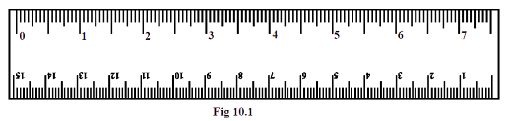Ans: The correct answer is (c) $0.2m$

Explanation: $0.2m$ cannot be measured by using given scale only once because measuring scale is only $0.15m$ long.

4. A piece of ribbon folded five times is placed along a $30cm$ long measuring scale as shown in Fig. $10.2$.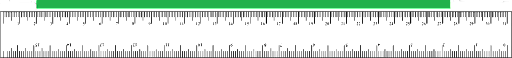The length of the ribbon is between

a) $1.15m - 1.25m$

b) $1.25m - 1.35m$

c) $1.50m - 1.60m$

d) $1.60m - 1.70m$

Ans: The correct answer is (b) $1.25m - 1.35m$.

Explanation: The length of five times folded ribbon = $\left( {27.5 - 2} \right)cm \times 5$

$= 1.275m$

Which lies between $1.25m - 1.35m$.

5. Paheli moves on a straight road from point A to point C. She takes $20$ minutes to cover a certain distance AB and $30$ minutes to cover the rest of distance BC. She then turns back and takes $30$ minutes to cover the distance CB and $20$ minutes to cover the rest of the distance to her starting point. She makes $5$ rounds on the road the same way. Paheli concludes that her motion is

a) Only rectilinear motion.

b) Only periodic motion.

c) Rectilinear and periodic both.

d) Neither rectilinear nor periodic.

Ans: The correct answer is (c) rectilinear and periodic both.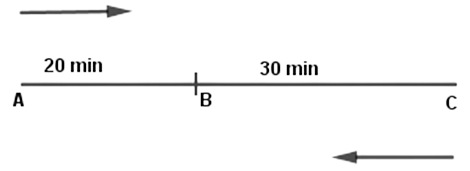Explanation: Paheli is moving along a straight line, so her motion is rectilinear and she is also moving with a fixed time period, so her motion is periodic. Therefore, the correct answer is both rectilinear and periodic.

6. Bholu and Golu are playing in a ground. They start running from the same point A in the ground and reach point B at the same time by following the paths marked $1$ and $2$ respectively as shown in Fig. $10.3$. Which of the following is/are true for the given situation.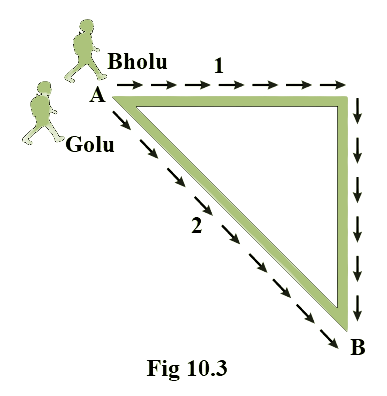As compared to Golu, Bholu covers a

a) Longer distance but with a lower speed.

b) Longer distance with a higher speed.

c) Shorter distance with a lower speed.

d) Shorter distance with a higher speed.

Ans: The correct answer is (b) longer distance with a high speed.

Explanation: We can see in the given figure that path $1$ is longer than $2$, but both have reached point B at the same time. So, Bholu walked faster than Golu.

7. Four pieces of wooden sticks A, B, C and D are placed along the length of $30cm$ long scale as shown in Fig. $10.4$. Which one of them is $3.4cm$ in length?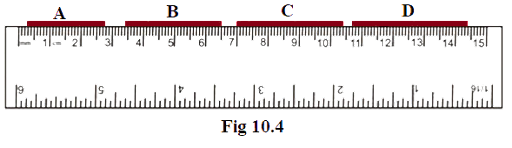a) A

b) B

c) A

d) D

Ans: The correct answer is option (c) C.

Explanation: Length of a wooden stick = Final reading of scale – Initial reading of scale.

 Name of wooden stick Length of a wooden stick = Final reading of scale – Initial reading of scale. A $2.7 - 0.3 = 2.4cm$ B $6.5 - 3.4 = 3.1cm$ C $10.4 - 7 = 3.4cm$ D $14.4 - 10.7 = 3.7cm$

8. Which of the following figure shows the correct placement of a block along a scale for measuring its length?

a)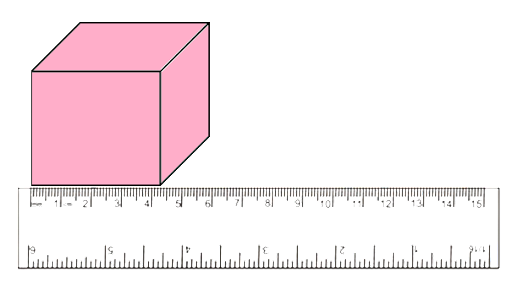b)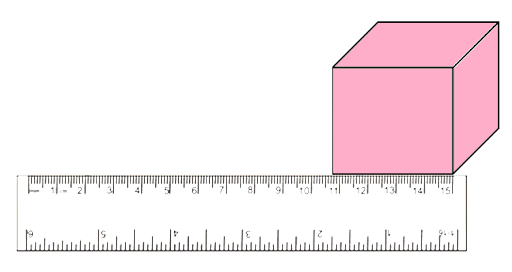c)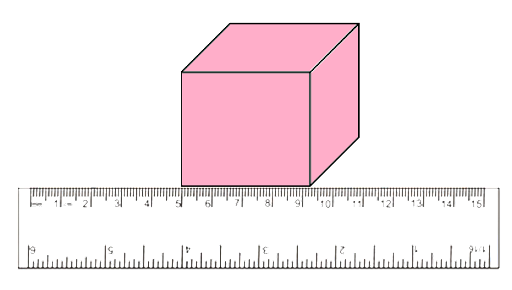d)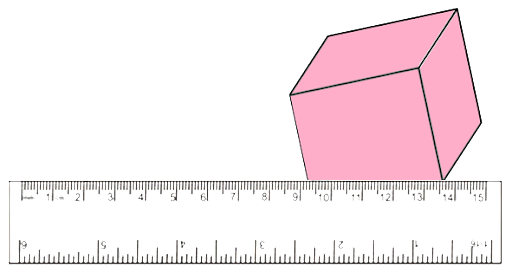Ans: The correct answer is (c).

Explanation: Object should be placed on a scale in such a way that its vertices lie in between the readings marked on the scale.

9. You are provided three scales A, B and C as shown in Fig. $10.6$ to measure a length of $10cm$.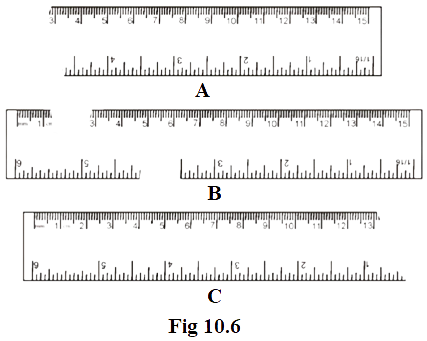For the correct measurement of the length you will use the scale

a) A only

b) B only

c) C only

d) Any of the three scales

Ans: The correct answer is (d) any of the three scales.

Explanation: Length of $10cm$ is available in all the scales.

10. Correct the following.

i. The motion of a swing is an example of rectilinear motion.

Ans: During the movement of swing periodic motion is shown rather than rectilinear motion. As, the swing is repeating its motion after a certain period of time.

ii. $1m = 1000cm$

Ans: A cm is a $\dfrac{1}{{100}}th$ part of a metre. So, $1m = 100cm$

11. Fill in the blanks

i. Motion of an object or a part of it around a fixed point is known as ................ motion.

Ans: Circular

ii. A body repeating its motion after certain interval of time is in ........... motion.

Ans: Periodic

iii. In rectilinear motion, object moves........... a ............ line.

Ans: Along; straight

iv. SI unit of length is ............

Ans: Metre

12. Write one example for each of the following type of motion.

i. Rectilinear

ii. Circular

Ans: Moving blades of a fan.

iii. Periodic

Ans: Motion of a swing.

iv. Circular and periodic

Ans: Tip of minute hand of a clock.

13. The photograph given as Fig. $10.7$ shows a section of a grille made up of straight and curved iron bars. How would you measure the length of the bars of this section, so that the payment could be made to the contractor?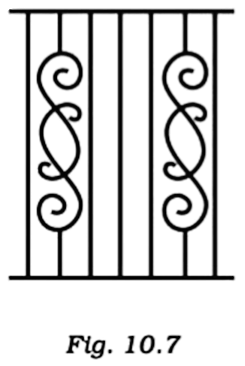Ans: We can see in the given figure that the grille is made up of two types of iron bars, straight and curved. We can use a measuring tape to measure straight iron bars and to measure curved iron bars, we can use thread first and then we can evaluate the result on a scale.

14. Identify the different types of motion in the following word diagram given as Fig. $10.8$.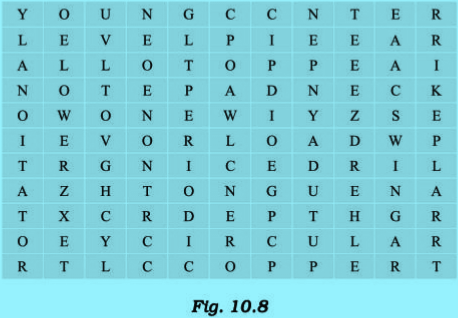Ans: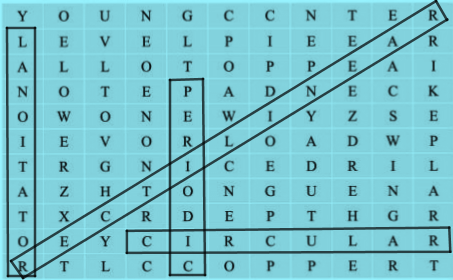15. Four children measure the length of a table which was about $2m$. Each of them used different ways to measure it.

i. Sam measured it with a half metre long thread.

ii. Gurmeet measured it with a $15cm$ scale from her geometry

iii. Reena measured it using her hand span.

iv. Salim measured it using a $5m$ long measuring tape.

Which one of them would get the most accurate length? Give reason for your answer.

Ans: Option (iv) is the correct answer.

Explanation: As Salim is using a standard instrument that is a $5m$ long measuring tape. The measurement will be accurate and it will be easy for Salim to measure the table quickly.

16. Match the events related to motion in Column I with the types of motions given in Column II.

 Column I Column II a) A moving swing. i. Periodic motion b) A movement of tip of the minute hand of a clock in one hour. ii. Rotational motion c) A moving wheel of a sewing machine. iii. Circular motion

Ans: The correct match is given below:

 Column I Column II a) A moving swing. ii. Rotational motion b) A movement of tip of the minute hand of a clock in one hour. iii. Circular motion c) A moving wheel of a sewing machine. i. Periodic motion

17. While travelling in a train, it appears that the trees near the track are moving whereas co-passengers appear to be stationary. Explain the reason.

Ans: The trees near the track appear to be moving in direction opposite to us because the position of these trees keeps changing with respect to us. Whereas, the position of our co-passengers doesn’t changes with respect to us and that is why they seem to be stationary.

18. How are the motions of a wheel of a moving bicycle and a mark on the blade of a moving electric fan different? Explain.

Ans: When the wheels of a bicycle start rotating, the bicycle moves forward (unless we apply breaks). So we can see both circular and rectilinear motion in the wheels of a bicycle. On the other hand, only circular motion can be seen when fan blades are in motion as the movement of blades doesn’t changes the position of the fan.

19. Three students measured the length of a corridor and reported their measurements. The values of their measurements were different. What could be the reason for difference in their measurements? (Mention any three)

Ans: Reasons for difference in their measurements could be-

1. Non standardized instrument.

2. Wrong method of measurement.

3. Use of different scales or devices.

20. Boojho was riding in his bicycle along a straight road. He classified the motion of various parts of the bicycle as

i. Rectilinear motion

ii. Circular motion and

iii. Both rectilinear as well as circular motion.

Can you list one part of the bicycle for each type of motion? Support your answer with reason.

Ans:

 S. No. Type of motion Part of bicycle Reason i. Rectilinear Handles Rectilinear motion is observed during the movement of handles. ii. Circular Pedals The pedals cannot move in any other direction except circular because they are fixed to a chain. iii. Both rectilinear and circular Wheels When the wheel rotates the bicycle moves forward too. So, rectilinear and circular motions are both shown in the wheels of a moving bicycle.

Motion and Measurement of Distances – Class 6, Science, Chapter 10 (CBSE)

In this chapter, students will learn about motion and ways to measure distances using different formulas. Measurements, SI units of measurement, concepts related to motions will be discussed in this chapter. Motion in a straight line, circular and rectilinear motion, and periodic motion will be learned by the students. This will be an introduction to all the important concepts of physics which will be helpful for students in their higher classes.

The NCERT Exemplar provided by Vedantu will be beneficial for students as it has all the necessary notes, revision notes, solved questions, and explanations. This will help students in their studies who can use it as a reference and for revision before their examinations. Students can download the files which are as PDF from the Vedantu platforms (app and website) and start learning. In addition, if there are any doubts and students need help with something then they may take the help of the Vedantu subject experts who will be a great guide in clearing doubts as well as for making concepts more clear.

## FAQs on NCERT Exemplar for Class 6 Science Solutions Chapter 10 Motion and Measurement of Distances

1. What is the measurement of the area?

Area can be defined as the space or amount of surface occupied by an object or someplace. The area can be calculated using different formulas for different surfaces or shapes. It is calculated by multiplying the length and breadth. It can be written as:

The SI unit for area is square meters represented as m2. Some other units are cm2, km2 and mm2. These are usually for smaller area measurements. The larger areas are measured using acre or hectare. For example,1 acre= 100 m2. For measuring irregular surfaces, graph papers are used as there are no formulas for finding such shapes’ areas.

2. How is volume measured?

Volume can be defined as the space or area that has been occupied by a substance which can be in a solid, liquid, or gas state. It is usually given by multiplying length, breadth, and height (length x breadth x height = Volume). The SI unit of volume is a cubic meter which is written as m3. The CGS standard unit for volume is a centimeter cube written as cm3 . The volume for irregular bodies cannot be measured using this formula. Some volumes for bodies that contain liquid can be measured directly. For example, a measuring jar is used to measure liquids that can be used directly for the required amount. Similarly, measuring vessels are used to measure the volume of liquids like oil and milk.

3. How is time measured?

Time can be defined as the interval between two different events. The SI unit for it is second (s). There are different units for a time that are used accordingly. For 1 minute, the total amount of seconds is 60. That means 60 seconds makes 1 minute. Similarly, 1 hour contains 60 minutes. A day has 24 hours. Time is measured in days too like in a year, we have 365 days and a decade means 10 years. Thus, these are some of the ways time can be measured.

4. What are rest and motion?

Rest is the state where a body or object is not in motion or moving. This is if the object doesn’t change its position concerning the reference point. This object which is not moving (i.e. stationary) is said to be at rest. For example, the table in your room is in a rest state compared to your room. On the other hand, motion is the opposite of rest which means the body or object is moving from one place to another and it is not stationary. For example, a vehicle that is moving or raindrops that fall on the ground due to the gravitational pull of the earth.

5. Can I get free NCERT Solutions for Chapter 10 of Science Class 6?

Yes, you can get all the NCERT solutions for this chapter. You can download them on the Vedantu platforms (app and website) by simply logging in or signing up. These solutions are free of cost and will help you with your studies. They are prepared by our Vedantu tutors who have years of experience in teaching and are subject experts who have prepared the solutions with explanations that will help you understand the concepts better.